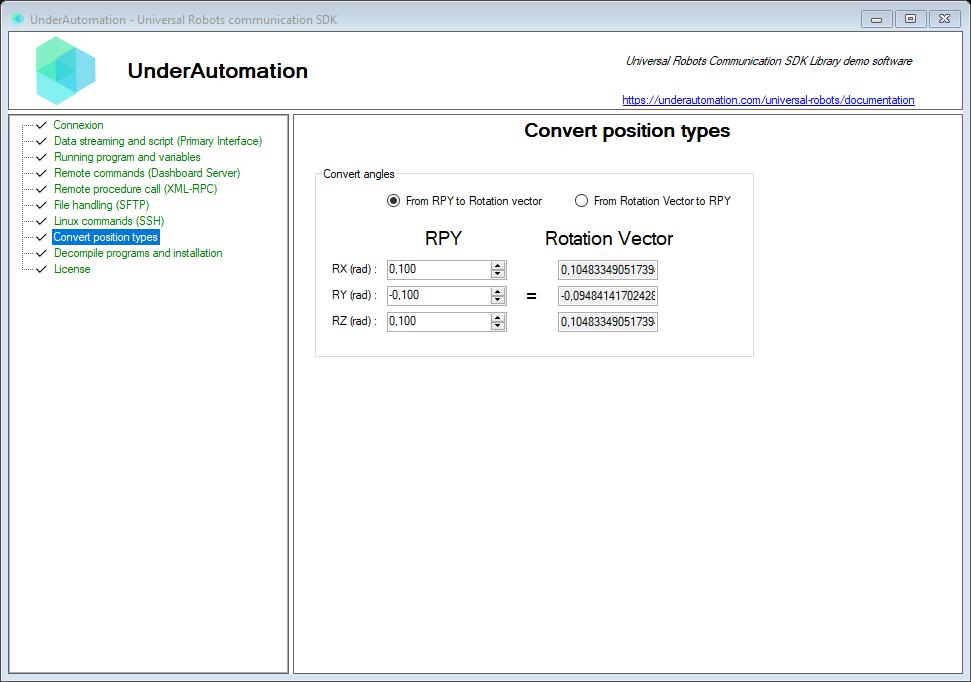# Convert position types

## Overview

The class `Pose` contains the 6 coordinates of a cartesian position : 3 translations X, Y, Z in millimeters and 3 rotation RX, RY, RZ in radians. It also contains 3 rotations properties that exposes the 3 rotations in degrees, but it's not a storage, only a conversion of RX, RY and RZ.

The methods `FromRotationVectorToRPY()` and `FromRPYToRotationVector()` transform the position in a new position with same translations X, Y, Z but different rotations.

A `Pose` instance can be returned in a XML-RPC answer. It will be interpreted as a robot pose.

Members of Common.Pose :
`public class Pose : CartesianCoordinates {     public Pose()     public Pose(double x, double y, double z)     public Pose(double x, double y, double z, double rx, double ry, double rz)     // Consider this pose as a Rotation Vector And convert it to a new RPY position    public Pose FromRotationVectorToRPY()     // Consider this pose as RPY And convert it to a new Rotation Vector    public Pose FromRPYToRotationVector()     // RX rotation in degrees or °/s    public double RxDegrees { get; set; }     // RY rotation in degrees or °/s    public double RyDegrees { get; set; }     // RZ rotation in degrees or °/s    public double RzDegrees { get; set; }     // Returns 6 comma separated coordinated with G1 format    public override string ToString()     // Pase a pose from its string representation    public static bool TryParse(string value, out Pose pose)}`

## Try it with the Windows example